Clocks

How long track travel the second hand in 46 hours, the end of which is 4 cm long.

Result

x =  693.66 m

Solution:Leave us a comment of example and its solution (i.e. if it is still somewhat unclear...):Be the first to comment!To solve this example are needed these knowledge from mathematics:

Do you want to convert length units?

Next similar examples:

1. Clock handsThe second hand has a length of 1.5 cm. How long does the endpoint of this hand travel in one day?
2. Minute-hand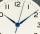How long distance will travel a large (minute) hand on the clock for 47 minutes, if its length is 43 cm?
3. Athlete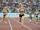How long length run athlete when the track is circular shape of radius 120 meters and an athlete runs five times in the circuit?
4. Circle - simpleThe circumference of a circle is 930 mm. How long in mm is its diameter?
5. Little hand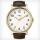What angle shifted little hand on the clock after one hour and 38 minutes?
6. MineWheel in traction tower has a diameter 5 m. How many meters will perform an elevator cabin if wheel rotates in the same direction 49 times?
7. Wheels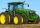Small tractor wheel with a diameter of 60 cm must be rotated 8 times to overcome some pathway. How many times must turn the big tractor wheel with a radius 60 cm to overcome the same distance?
8. FlowerbedIn the park there is a large circular flowerbed with a diameter of 12 m. Jakub circulated him ten times and the smaller Vojtoseven times. How many meters each went by and how many meters did Jakub run more than Vojta?
9. CircleWhat is the radius of the circle whose perimeter is 6 cm?
10. Bicycle wheelAfter driving 157 m bicycle wheel rotates 100 times. What is the radius of the wheel in cm?
11. WellRope with a bucket is fixed on the shaft with the wheel. The shaft has a diameter 50 cm. How many meters will drop bucket when the wheels turn 15 times?
12. Two circlesTwo circles with a radius 4 cm and 3 cm have a center distance 0.5cm. How many common points have these circles?
13. Circle r,DCalculate the diameter and radius of the circle if it has length 52.45 cm.
14. Ferris wheel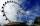Ferris wheel reaches to 22 m tall and moves at the speed of 0.5m/s. During one drive wheel rotates three times. What is the total drive time?
15. Two gears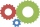The two gears fit together. The larger gear has 32 teeth, the smaller has 20 teeth less. How many times does turn a smaller gear if the bigger gear turns three times?
16. Pulley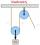On wheels with a diameter of 40 cm is fixed rope with the load. Calculate how far is load lifted when the wheel turns 7 times?
17. BaseBase of building is circle with diameter 25 m. Calculate the circumference of a circular trench witch diameter is 41 cm wider than the diameter of the base.